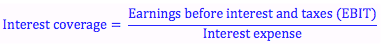Explore BrainMass

# Interest Coverage

The interest coverage ratio (or times-interest-earned ratio) is calculated by dividing earnings before interest and taxes by the interest expense. It gives us an idea about how well the firm is able to generate earnings to cover its interest expenses.We use the earnings before interest and taxes to calculate the interest coverage ratio. We want earnings before interest is paid because it reflects the total amount of earnings available to both debt holders and shareholders. Earnings after interest was paid would reflect only those earnings available to shareholders. We could calculate the ratio of earnings paid to shareholders and earnings paid to debt holders (interest expense), but that’s not what this ratio is for. (We also do before taxes because a firm’s tax expense relies on how much interest was paid. If interest expense = EBIT, then no taxes would be paid).

Here we want to know how much greater our total earnings are then the amount of fixed payments we have to make to debt holders. This gives us information about the solvency of the firm – are we able to make these payments out of earnings easily?